# Multiplying and dividing rational expressions 2 | Algebra II | Khan Academy

##By Khan Academy

Khan Academy presents Multiplying and Dividing Rational Expressions 2, an educational video resource on math.# Expressing division in multiple ways

##By Khan,Academy

This word problem is solved by showing 4 different ways to divide 18 by 2.# Complex Numbers: Multiplying and Dividing in Polar Form, Ex 2

##By PatrickJMT

Complex Numbers: Multiplying and Dividing in Polar Form, Ex 2. In this video, I give the formula for multiplication and division of two complex numbers that are in polar form.# How to divide positive and negative signed rational numbers (7.NS.2.b)

##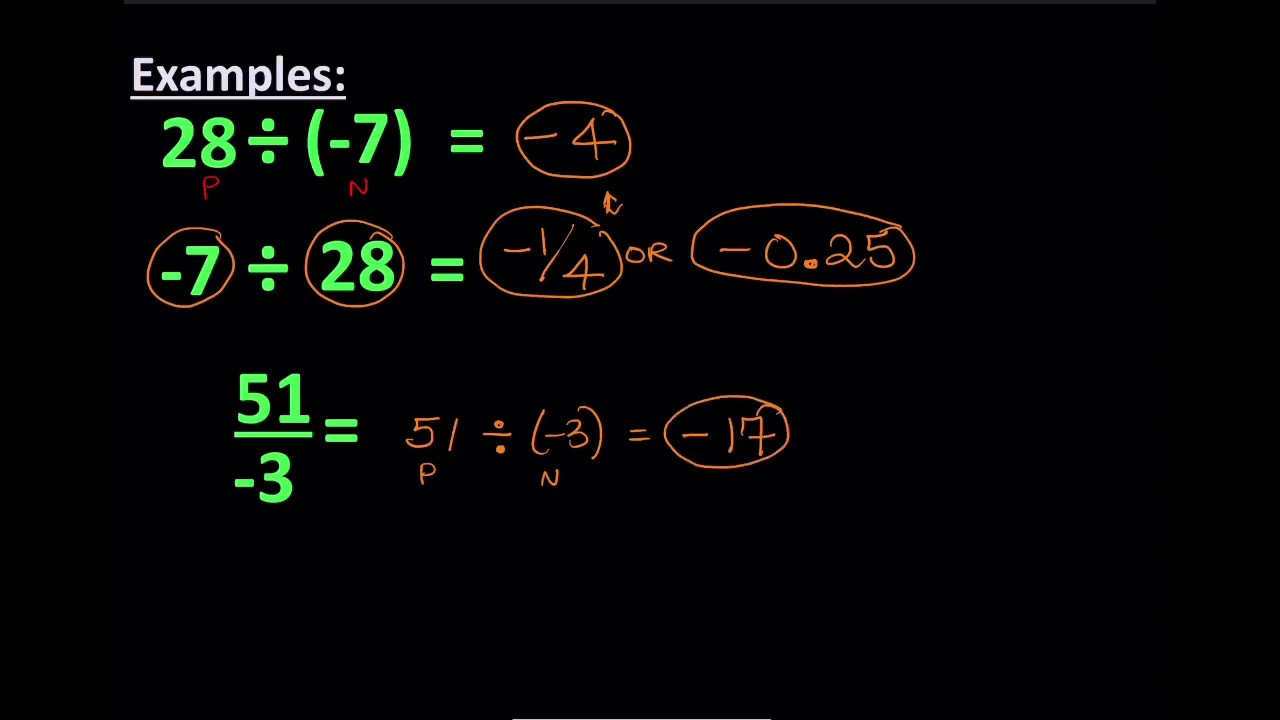By Rob Oliver

This lesson covers how to divide positively and negatively signed rational numbers. This covers CC standard: 7.NS.2.b# Divide polynomials by monomials with remainders

##By Khan Academy

Sal divides (18x^4-3x^2+6x-4) by 6x.# Dividing decimals 2

##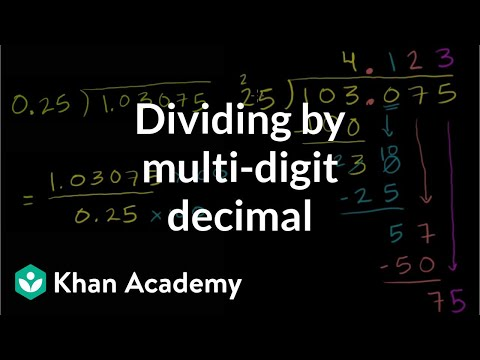By Khan Academy

Let's do one more decimal division problem together but change it up. This is one with a decimal divisor and will require you to shift the decimal first.# DEPRECATED Dividing polynomials by binomials 2

##By Khan Academy

Salï¿½ï¿½ï¿½ï¿½ï¿½ï¿½ï¿½ï¿½ï¿½ï¿½ï¿½ï¿½simplifies and states the domain of (2x^2+13x+20)/(2x^2+17x+30).# DEPRECATED Dividing polynomials by binomials 2

##By Khan Academy

Sal explains what it means to simplify a rational expression and why we would want to do that. Just don't forget the excluded values!# DEPRECATED Dividing polynomials by binomials 2

##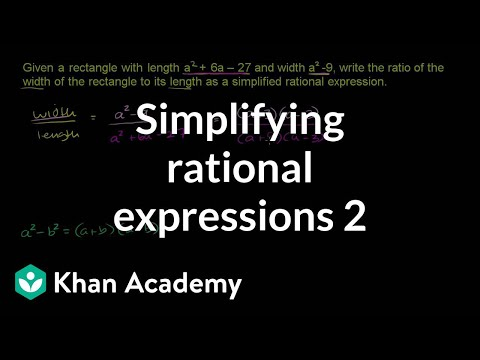By Khan Academy

Sal solves the following problem:ï¿½ï¿½ï¿½ï¿½ï¿½ï¿½ï¿½ï¿½ï¿½ï¿½ï¿½ï¿½Given a rectangle with length a^2+6a+27 and width a^2-9, write the ratio of the width to the length as a simplified rational expression.# Divide polynomials by monomials with remainders

##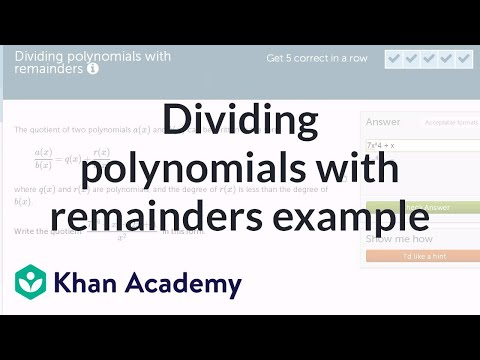By Khan Academy

Sal divides (7x^6+x^3+2x+1) by X^2, and writes the solution as q(x)+r(x)/x^2, where the degree of the remainder, r(x), is less than the degree of x^2.# Divide polynomials by linear binomials with remainders

##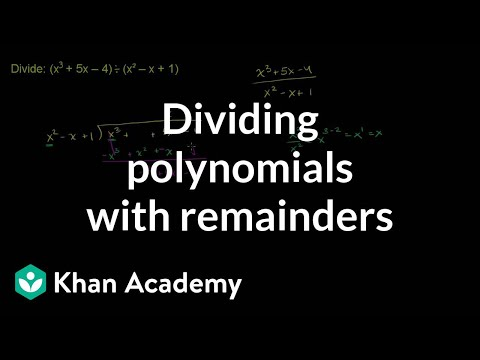By Khan Academy

Sal divides (x^3+5x-4) by (x^2-x+1) using long division.# Dividing decimals 2

##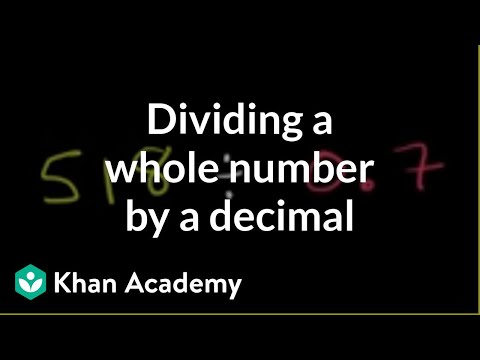By Khan Academy

When dividing a whole number by a decimal, you're going to need to change that decimal into a whole number. How? We'll show you.# Dividing decimals 2

##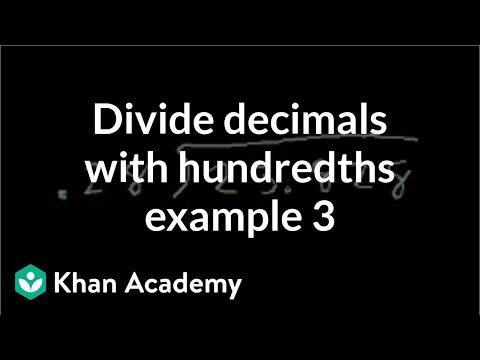By Khan Academy

The old saying is practice makes perfect. Let's keep practicing dividing decimals and making our divisor a whole number.# Polynomials

##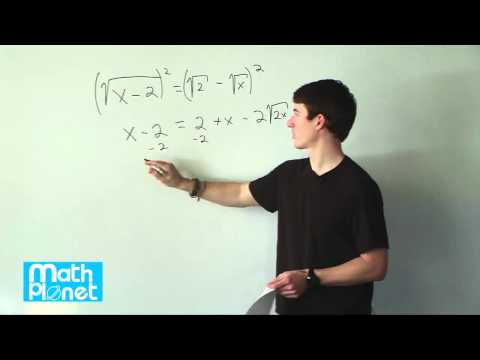By MathPlanetVideos

Divide x2-2x-8 by x+2 (same as the example above).# Area models to visualize division using place value | 4th grade | Khan Academy

##By Khan Academy

We can break up big division problems into smaller, easier problems. In this video, we solve 268 divided by 2 and 856 divided by 8.# [5.NBT.2-2.0] Multiplying/Dividing by 10 - Common Core Standard

##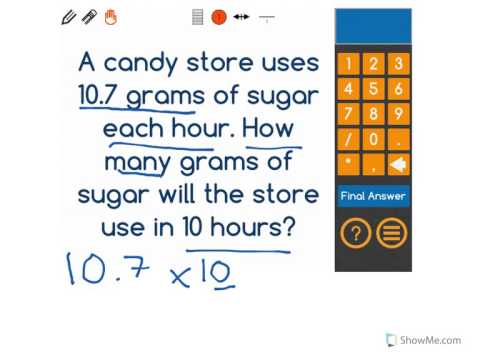By Front Row

Discover more Common Core Math at https://www.frontrowed.com Explain patterns in the placement of the decimal point when a decimal is multiplied by a power of 10, use whole-number exponents to denote powers of 10 Front Row is a free, adaptive, Common Core aligned math program for teachers and students in kindergarten through eighth grade. Front Row allows students to practice math at their own pace - learning advanced concepts when they're ready and receiving remediation when they struggle. Front Row provides teachers with access to a detailed data dashboard and weekly email reports that show which standards are causing students difficulty, what small groups can be formed for interventions, and how their students are progressing in math.Discover more Common Core Math at https://www.frontrowed.com# [5.NBT.2-3.0] Multiplying/Dividing by 10 - Common Core Standard

##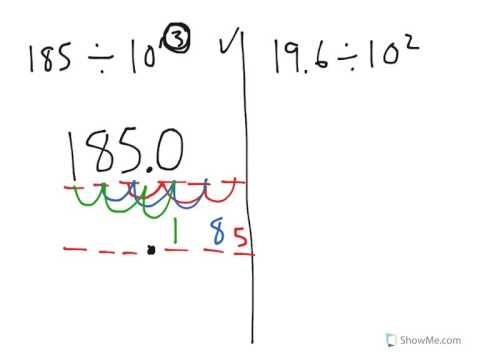By Front Row

Discover more Common Core Math at https://www.frontrowed.comExplain patterns in the placement of the decimal point when a decimal is divided by a power of 10, use whole-number exponents to denote powers of 10Front Row is a free, adaptive, Common Core aligned math program for teachers and students in kindergarten through eighth grade. Front Row allows students to practice math at their own pace - learning advanced concepts when they're ready and receiving remediation when they struggle. Front Row provides teachers with access to a detailed data dashboard and weekly email reports that show which standards are causing students difficulty, what small groups can be formed for interventions, and how their students are progressing in math.Discover more Common Core Math at https://www.frontrowed.com# Use the Polynomial Remainder Theorem to analyze factors of polynomials

##By Khan Academy

Sal finds the remainder of (-3x^3-4x^2+10x-7) divided by (x-2) using the PRT (Polynomial Remainder Theorem).# Dividing Polynomials

##By Carter Maslan

step-by-step example of dividing the polynomial (x^2 + 3x - 40) / (x - 5).# Divide polynomials by polynomials with remainders

##By Khan Academy

Sal divides (x^3+5x-4) by (x^2-x+1) using long division.